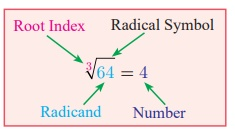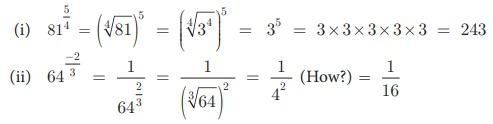Home | | Maths 9th std | Radical Notation

## Chapter: 9th Maths : UNIT 2 : Real Numbers

The symbol n√ (read as nth root) is called a radical; n is the index of the radical (hitherto we named it as exponent); and x is called the radicand.

Let n be a positive integer and r be a real number. If rn = x, then r is called the nth root of x and we write

n√x = r

The symbol n (read as nth root) is called a radical; n is the index of the radical (hitherto we named it as exponent); and x is called the radicand.

Note

It is worth spending some time on the concepts of the ‘square root’ and the ‘cube root’, for better understanding of surds.

What happens when n = 2? Then we get r2 = x, so that r is 2x, our good old friend, the square root of x. Thus 2√16 is written as √16 , and when n =3, we get the cube root of x, namely 3x . For example, 3√8 is cube root of 8, giving 2. (Is not 8 = 23?)

How many square roots are there for 4? Since (+2) × (+2) = 4 and also (–2)×(–2) = 4, we can say that both +2 and –2 are square roots of 4. But it is incorrect to write that √4 = ± 2 .

This is because, when n is even, it is an accepted convention to reserve the symbol nx for the positive nth root and to denote the negative nth root by – nx. Therefore we need to write √4 = 2 and −√4 = −2.

When n is odd, for any value of x, there is exactly one real nth root. For example, 3√8 = 2 and 5√−32 = −2.

### Thinking Corner

Which one of the following is false?

(1) The square root of 9 is 3 or –3.

(2) √9 = 3

(3) −√9 = −3

(4) √9 = ±3

## 1. Fractional Index

Consider again results of the form r = nx.

In the adjacent notation, the index of the radical (namely n which is 3 here) tells you how many times the answer (that is 4) must be multiplied with itself to yield the radicand.To express the powers and roots, there is one more way of representation. It involves the use of fractional indices.

We write nx as x1/n

With this notation, for example

3√64 is 641/3 and √25 is 251/2.

Observe in the following table just some representative patterns arising out of this new acquaintance:Example 2.16

Express the following in the form 2n :

(i) 8 (ii) 32 (iii) 1/4 (iv) √2 (v) √8.

Solution

(i) 8 = 2 ×2 × 2 ; therefore 8 = 23

(ii) 32 = 2×2×2×2×2 = 25

(iii) 1/4 = 1/(2×2) = 1/22 = 2−2

(iv) √2 = 21/2

(v) √8 = √2×√2×√2 = (21/2)3 which may be written as 23/2

Meaning of xm/ n, (where m and n are Positive Integers)

We interpret xm/n either as the nth root of the mth power of x or as the mth power of the nth root of x.

In symbols, xm/n = (xm)1/n or (x1/n)m = nxm or (nx)m

Example 2.17

Find the value of (i) 815/4 (ii) 64-2/3

SolutionTags : Real Numbers | Maths , 9th Maths : UNIT 2 : Real Numbers
Study Material, Lecturing Notes, Assignment, Reference, Wiki description explanation, brief detail
9th Maths : UNIT 2 : Real Numbers : Radical Notation | Real Numbers | Maths